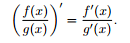# FDQR ( Freshman Dream Quotient Rule)

## Homework Statement

I have been working with researching and writing a paper on the Freshman Dream Quotient Rule. This rule states, and I was wondering if anyone can come up with an example of 2 functions which make this work.

## The Attempt at a Solution

I have looked at proofs and everything of this, but have not been able to come up with an example for my paper. For all I know, there may not be an example.

stevendaryl
Staff Emeritus
You can use the laws of derivatives to write:

$(\frac{f}{g})' = \frac{f'}{g} - \frac{f g'}{g^2}$

So you're trying to solve:
$\frac{f'}{g} - \frac{f g'}{g^2} = \frac{f'}{g'}$

or multiplying through by $g^2 g'$:

$f' g g' - f (g')^2 = f' g^2$

We can separate the $f$ and $g$ be rewriting as;

$f'(g g' - g^2) = f (g')^2 \Rightarrow \frac{f'}{f} = \frac{(g')^2}{g g' - g^2} = \frac{g'}{g} + \frac{g'}{g' - g}$

So try picking some simple function for $g$ and seeing if you can solve for $f$.

pasmith
Homework Helper
If $f(x) = e^{kx}$, $g(x) = e^{lx}$ then $$\left(\frac{f}{g}\right)' = (k - l)e^{(k-l)x}, \\ \frac{f'}{g'} = \frac kl e^{(k-l)x}.$$ These can be made equal by suitable choice of $k$ and $l$.

•mfb
mfb
Mentor
$\frac{(g')^2}{g g' - g^2} = \frac{g'}{g} + \frac{g'}{g' - g}$
That looks like a freshman's dream on its own but it is correct.
That approach leads to a nice solution for some simple polynomial g.